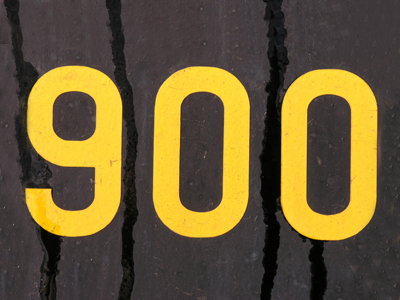In 900 the 9 is in the hundreds' position.

# Place Value 2 (Very Easy)

This is the second of our very easy Eleven Plus maths quizzes on place value. Like the first, it will ask you to find the numbers in the thousands, hundreds, tens, and ones positions. This quiz, and its fellows, serve as an introduction to the topic before we pose you some harder questions later on.

As you will know, the value of a digit in a number depends on its position. For example, a 2 could represent 2, 20, 200 or even 2,000, depending on where it is placed.

The rules of place value are quite simple: starting from the right, the first position is ones (times number by 1), the second is tens (times number by 10), the third is hundreds (times number by 100), and the fourth is thousands (times number by 1,000).

There are other values, like tenths, hundredths, ten-thousands and more, but we’ll save these for later quizzes. For now, have a go at this simple quiz. It shouldn’t be too much of a problem.

1.
What is the digit in the tens’ place in 8,562?
8
5
6
2
Reading the number from right to left: the 6 is in the tens’ place
2.
What is the digit in the tens' place in 2,103?
2
1
0
3
Reading the number from right to left: the 0 is in the tens' place
3.
What is the place value of 8 in 8,309?
The ones' place
The tens' place
The hundreds' place
The thousands' place
Reading the number from right to left: 8 is in the thousands’ place
4.
What is the place value of 7 in 7,024?
The ones' place
The tens' place
The hundreds' place
The thousands' place
Reading the number from right to left: 7 is in the thousands’ place
5.
What is the digit in the tens' place in 1,832?
1
8
3
2
Reading the number from right to left: the 3 is in the tens' place
6.
What is the digit of the thousands’ place in 9,023?
9
0
2
3
Reading the number from right to left: 9 is in the thousands’ place
7.
What is the digit in the thousands' place in 5,290?
5
2
9
0
Reading the number from right to left: the 5 is in the thousands' place
8.
What is the place value of 5 in 8,453?
The ones' place
The tens' place
The hundreds' place
The thousands' place
Reading the number from right to left: 5 is in the tens’ place
9.
What is the place value of 4 in 2,094?
The ones' place
The tens' place
The hundreds' place
The thousands' place
Reading the number from right to left: 4 is in the ones’ place
10.
What is the place value of 7 in 1,372?
The ones' place
The tens' place
The hundreds' place
The thousands' place
Reading the number from right to left: 7 is in the tens' place
Author:  Frank Evans